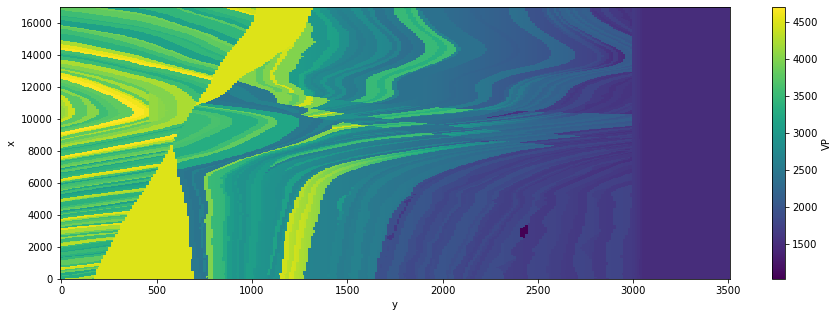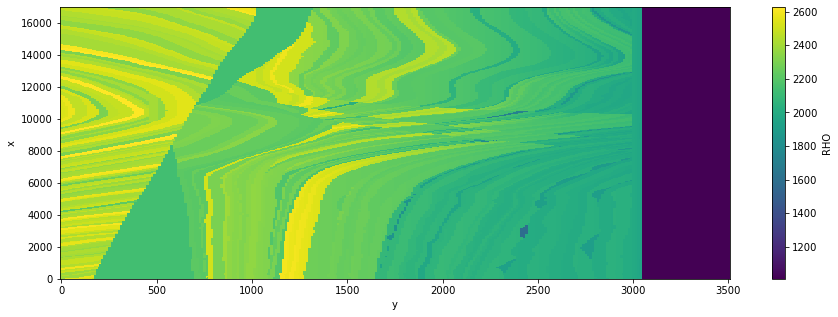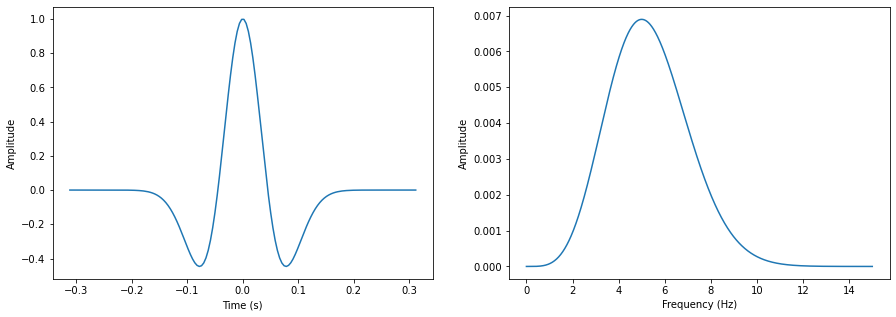Version:

This documentation is not for the latest stable Salvus version.This tutorial is presented as Python code running inside a Jupyter Notebook, the recommended way to use Salvus. To run it yourself you can copy/type each individual cell or directly download the full notebook, including all required files.# Marmousi Model

## Introduction

The AGL Elastic Marmousi model is the most recent generation of the classic Marmousi seismic benchmark. In this tutorial we will cover how one can use this model within Salvus. In particular, we will cover:

• How to read a SEG-Y file using Obspy
• How to read in a rectilinear model using xarray
• How to interpolate a 2-D regularly-gridded model onto the mesh
• How to generate a synthetic seismic shotgather using the model

## Environment setup

As always, our first job is to import the relevant Python packages into our notebook.

Copy
%matplotlib inline
%config Completer.use_jedi = False

from pathlib import Path
import os
import requests
import shutil
from typing import List

import scipy
import obspy
import numpy as np
import xarray as xr
import matplotlib.pyplot as plt

from salvus.flow import api
from salvus.flow import simple_config as config

from salvus.toolbox import toolbox

SALVUS_FLOW_SITE_NAME = os.environ.get("SITE_NAME", "local")

## Model building

Information about the Marmousi model we'll be using can be found here. The model is stored as a SEG-Y file, and the next cell will download and unpack the model for you. Note that the model file is relatively large (~150 MB), so make sure you have enough disk space before continuing.

# Download Marmousi model ~150 MB.
target_path = "elastic-marmousi-model.tar.gz"
if not Path(target_path).exists():
url = (
"https://s3.amazonaws.com/open.source.geoscience/open_data"
"/elastic-marmousi/elastic-marmousi-model.tar.gz"
)

response = requests.get(url, stream=True)
if response.status_code == 200:
with open(target_path, "wb") as f:
shutil.unpack_archive(target_path)

With our environment is set up, and the model downloaded, we can begin building the Salvus mesh. Most material models in seismic exploration are discretized onto a regular grid, and the open-source SalvusPythonToolkit provides a simple interface to create two or three dimensional Salvus meshes based on such models. As models can be stored in a wide variety of format such as SEG-Y, or RSF, we use xarray as an intermediary to encapsulate both the model parameters and geometry. More info on xarray, including extensive documentation, can be found here. Reading our Marmousi model into an xarray.Dataset is trivial, and the process can be inspected by opening the acompanying getting_started_tools.py file.

In general, if you are working working with a regularly gridded model in either two or three dimensions, a recipie similar to the function provided should be all you need to do to get your model into a form Salvus Mesh can understand. All that is required is an xarray dataset with the following information

• The coordinate values in meters (named 'x', 'y', and optionally 'z')
• The material parameters in SI units, with names following the parameter naming convention
# Read marmousi model.
model_directory = Path("./elastic-marmousi-model/model")
marmousi_model = toolbox.read_elastic_marmousi(model_directory, ["VP", "RHO"])

A nice feature of xarray.Dataset object is the ability to quickly plot the contained data. Let's do this below as a way to quickly QC that the model reading went correctly.

marmousi_model["VP"].plot(aspect="auto", figsize=(15, 5))
plt.show()
marmousi_model["RHO"].plot(aspect="auto", figsize=(15, 5))
plt.show()Yup, all looks good!

### Meshing parameters

#### Frequency content

When building material models for use with wave propagation solvers such as Salvus, there are a few factors one needs to consider. Obviously we need to ensure that the spatial complexity of the model is adequetly captured by whatever spatial discretization we are choosing. What is less obvious however is that the details of the solver's spatial discretization are intimitely to both the spatial complexity of the model and the spatial complexity of the physical process we are solving for. When considering the propagation of seismic waves we are somewhat lucky that the solutions are band-limited and the spatial complexity of the solution can be reliably estimated before any simulations are run.

Using the simple formula $\lambda_{\text{min}} = v_{\text{min}} \cdot f_{\text{max}}^{-1}$, we have an estimate $\lambda_{\text{min}}$ for the minimum wavelength in our simulation when given the minimum velocity in the model ($v_{\text{min}}$) and the maximum frequency in the forcing function ($f_{\text{max}}$). It is this minimum wavelength, in conjuction with the spatial complexity of the model itself, which places the most restrictions on how we should build and discretize our model. No matter which numerical method we choose to solve the wave-equation we must have at least a certain number of "points" per minimum wavelength to ensure an accurate solution. In finite-difference (FD) simulations, these points are the discrete points of the FD grid. In spectral-element simulations (such as those performed by Salvus) these points are the GLL point locations with each spectral-element.

In Salvus, to properly balance accuracy and performance, we suggest to use anywhere from 6 - 9 points per minimum wavelength. When using standard 4th order elements (which have 5 GLL points along each edge), this equates to using 1.25 - 2 elements per wavelength. With this discussion in mind, one must first determine what frequency band they are interested in before one proceeds to the meshing stage, and this is why we now begin with the definition of our source wavelet. Below we choose a Ricker wavelet with a peak frequency of 1Hz, and plot the source-time function as well as the wavelet's frequency content.

wavelet = config.stf.Ricker(center_frequency=5.0)

f, ax = plt.subplots(1, 2, figsize=(15, 5))

ax.plot(*wavelet.get_stf())
ax.set_xlabel("Time (s)")
ax.set_ylabel("Amplitude")

ax.plot(*wavelet.get_power_spectrum())
ax.set_xlabel("Frequency (Hz)")
ax.set_ylabel("Amplitude")

plt.show()It's immediately obvious that the Ricker wavelet has a finite bandwidth, and that there is quite a lot of energy at frequencies higher than the center. It then follows that, if we're interested in accurately modelling the full bandwidth of the injected source, then we must chose $f_{\text{max}}$ to be higher than the center frequency of our wavelet. From experience we usually find, when considering a Ricker wavelet, that generating the mesh assuming a maximum frequency which is twice the central frequency provides a good balance between accuracy and performance. It is this rule which we will follow below.

#### Absorbing boundaries

To preserve the stability of the wavefield solution in the presence of complex or anisotropic media, Salvus employs a two-stage approach to absorbing boundaries. First, we apply absorbing boundary conditions at the edge of the mesh as outlined here. These conditions provide good absorbing characteristics for wave impacting the boundary at close to normal incidence, and are sufficient for most cases. If a more substantial absorbing profile is desired, one can also pad the simulated domain with a damping layer. This approach follows that given in this paper. Adding damping layers are advantageous in that they can almost completely cancel any boundary reflections, but do require one to enlarge the computational domain and therefore increase the cost of the resultant simulations. We have found that these boundaries, when using 4th order elements, perform best with 7 or more layers of elements. For the example below we will round this number up to 10. The type and performance of absorbing boundaries your application calls for will vary.

num_absorbing_layers = 10
absorbing_side_sets = ["x0", "x1", "y0"]

### Generating the mesh

With our model read in, and our frequency band and absorbing boundaries defined, we can now go ahead and generate the Salvus mesh itself. If you're not familiar with the concept of model order, please see the relevant tutorial here. For this model, due to the high spatial variability, we'll choose a 4th order model parameterization. Note here that we are setting the mesh_frequency parameter to twice the center frequency of the Ricker wavelet we will use, as described above.

mesh_frequency = 2 * wavelet.center_frequency
mesh = toolbox.mesh_from_xarray(
model_order=4,
data=marmousi_model,
slowest_velocity="VP",
maximum_frequency=mesh_frequency,
elements_per_wavelength=1.5,
absorbing_boundaries=(absorbing_side_sets, num_absorbing_layers),
)

The above function call should now have generated you mesh object. Note here that we are not applying any special meshing algorithms -- we'll get to those later. We're simply meshing the domain using a rectilinear mesh and assuming no surface topography, no sea-bottom topography, and no fluid / solid coupling.

### Visualizing the mesh

To visualize the mesh, it's now enough to just type mesh into the cell below. When run in a notebook, this command will plot an interactive 2- or 3-D plot of the simulation mesh, with the available parameters interpolated to the GLL points defining the model interpolation order. This is why we see variability within each element which is plotted below. You can expand the Parameter dialog box in order to visualize any parameter present in the mesh. Obviously, we have the spatially variable VP and RHO, which define 2-D acoustic parameters of our medium. In addition, we have the elemental-parameter fluid, along with absorbing_boundaries. The fluid flag is read by Salvus Compute and is used to determine which elements in the simulation are acoustic and which are elastic. In this case we see that fluid = 1 everywhere in the mesh, which is telling us that the entire mesh should simulation the fluid (acoustic) wave equation. In this tutorial and this tutorial we explore how to run coupled simulations, and see how one can change the fluid flag at will, and even vary it randomly between individual elements. The absorbing_boundaries flag simply shows us where the domain has been un-physically extended for use by the damping layers. This is handy to know, as we don't want to put sources or receivers in this area. As we specified ["x0", "x1", "y0"] as our absorbing side-sets, you should see the damping layers extending out of the left, bottom, and right sides of the mesh. Since we will be simulating a free-surface condition at the top of the model, no extension has been made here. As a reminder from earlier tutorials: you can visualize the side sets in the mesh by clicking the "Show Side Sets" button in the widget below.

mesh
<salvus.mesh.unstructured_mesh.UnstructuredMesh at 0x7fe7a8d2e350>

## Setting up a simulation

With the mesh generated, we can now go ahead and set up a WaveformSimulation object. This object encapsulates the parameters that we sent to Salvus Compute, and allows us to validate most of the parameters we pass before we begin a simulation. This helps to avoid any typos creeping into our final paramter files. We'll first need to finalize the definition of our source and define our receiver array.

#### Sources

Here we'll choose a scalar source type (as we're working in an acoustic medium), and use the side-set interface to allow for easy and intuitive source placement. Note that, since this is a purely acoustic simulation, if we chose an elastic source type (either Vector or MomentTensor) the simulation would give an error explaining that the given source type could not be attached to any element. We'll also reuse the stf object that we generated above for the purposes of mesh design. For more information on different source types, please see our documentation.

source = config.source.cartesian.ScalarPoint2D(
source_time_function=wavelet, x=8500.0, y=3490.0, f=1
)

Setting up receivers is similar to setting up sources. For this example we want to use a regularly-spaced grid of receivers which span the length of the domain. For this, we can use the SideSetHorizontalPointCollection2D receiver type. This allows us to choose a side-set (here, "y1"), and to place an array of receivers a certain distance from this side-set. In this case we choose to place the receivers 10 m below the ocean bottom. We also need to specify an array of dynamic fields to record. Here we choose "phi" and "gradient-of-phi", which can be related to pressure and particle velocity as outlined here. More information on receiver types can be found in our documentation.

receivers = config.receiver.cartesian.SideSetHorizontalPointCollection2D(
x=np.linspace(0.0, 17000.0, 1000),
offset=-10.0,
station_code="xx",
side_set_name="y1",
)

#### Parameters

To set up our simulation, we turn to the config.simulation.Waveform class. This class allows us to choose and validate many of the relevant simulation parameters. We'll first initialize the object by passing our mesh, along with the sources and receivers we defined above. You'll notice that when executing the following cell, a small progress bar appears and a set of "items" are "located". Here what is happening is that the receivers defined above are being placed exactly 10m away from the side-set "y1". Given that our domain is rectilinear and has no topography, this process is trivial, and we could of course have simply placed the receivers ourselves as we did for the source. However, once we add topography or bathmetry to our domain (to be outlined in a future tutorial), the automatic point placement can become essential for accurate source and receiver localization. For more information on attaching sources and receivers to deformed meshes, please see our documentation.

s = config.simulation.Waveform(mesh=mesh, sources=source, receivers=receivers)

Now with our simulation objects defined, we can set a few more parameters to customize our simulation. The first thing we'll do is set the timing parameters, namely the time_step_in_seconds, start_time_in_seconds, and end_time_in_seconds fields, which are part of the physics.wave_equation parameter group. If they are not set, Salvus will choose sensible defaults for all three of these parameters. The start and end times will default to the values needed to smoothly simulate the entire source wavelet, and the time step will be computed inside Salvus as per the CFL criterion. Here we set the end time to 8 seconds, and keep the rest of the parameters at their default values.

# Leave start time and time step as their auto-detected defaults.
# s.physics.wave_equation.start_time_in_seconds = ?
# s.physics.wave_equation.time_step_in_seconds = ?

# Set end time.
s.physics.wave_equation.end_time_in_seconds = 8.0

Now we'll set the boundary conditions. Below we choose a HomogeneousDirichlet condition for the top "y1" boundary. This corresponds to a pressure-free (or free-surface) condition in acoustic media. We'll also now use the absorbing boundary parameters we set above to mock an infinite domain extending from the other side-sets. As mentioned above, we've empirically found good results when the boundaries extend 7 or more elements from the edge of the physical domain, and the taper amplitude is equal to the center frequency of the chosen wavelet.

# Define Dirichlet boundary at top surface (pressure-free).
dirichlet = config.boundary.HomogeneousDirichlet(side_sets=["y1"])

# Define coupled Clayton-Enqist / Kosloff
# damping boundaries at the the remaining side-sets.
absorbing = config.boundary.Absorbing(
width_in_meters=685.333,
side_sets=["x0", "y0", "x1"],
taper_amplitude=wavelet.center_frequency,
)

# Add the boundaries to the parameter file.
s.physics.wave_equation.boundaries = [absorbing, dirichlet]

Currently Salvus is capable of outputing point (receiver) data in either the ASDF format, or a simplified format based on HDF5. While ASDF is a robust choice when performing regional or global-scale simulations, on the exploration scale the simplified HDF5 format should suffice. This format allows for the easy generation of shot gathers, as demonstrated in the final cell below.

s.output.point_data.format = "hdf5"

Finally, before we get everything running, we show below how one can specify volumetric (2- or 3-D) output for wavefield visualization and analysis. We don't output these by default because the file sizes involved can be quite large (in this case, the parameters below will produce 375 MB of output). If your machine has the capacity, feel free to comment the following in to generate time-varying volumetric output which can be visualized in Paraview.

# s.output.volume_data.format = "hdf5"
# s.output.volume_data.filename = "output.h5"
# s.output.volume_data.sampling_interval_in_time_steps = 100

## Running a simulation

#### Input file validation

Now that our mesh, source, receivers, and parameters are defined, we're ready to run our simulation. For a quick validation step, you can call .validate() on the simulation object. This will ensure that there are no typos in your parameter definitions, and that Salvus will accept the parameters and begin to run. It cannot catch all the possible errors which could occur (for example, if a source or receiver is placed outside of the domain, or if the simulation explodes due to a too-large time step), but it does help catch many common things before you set off a big job. For example, if you one of the fields in the volume_data.fields setting, the validator below would catch this.

s.validate()

#### Running on a Salvus Flow site

The simulation should take around 30 seconds on 2 cores, but performance will of course vary from machine to machine.

api.run(
input_file=s,
ranks=2,
site_name=SALVUS_FLOW_SITE_NAME,
get_all=True,
output_folder="output",
overwrite=False,
)
Job job_2012031852893819_6151f22600 running on local with 2 rank(s).
Site information:
* Salvus version: 0.11.23
* Floating point size: 32


* Downloaded 66.6 MB of results to output.
* Total run time: 39.37 seconds.
* Pure simulation time: 38.66 seconds.

<salvus.flow.sites.salvus_job.SalvusJob at 0x7fe7a8153650>

#### Visualizing output

When the simulation finishes, you should see a folder called output in your working directory. All of the files generated during the simulation copied there. If you chose to output volumetric data, you can simply open the .xdmf file in this output directory to visualize the wavefield in Paraview. Otherwise, you can use the included salvus.toolbox module to generate a shotgather directly from the output file as shown below.

# Generate a shotgather from and HDF5 receiver file.
data, dt, extent = toolbox.get_shotgather("output/receivers.h5", field="phi")

# Normalize and plot the shotgather.
clip_min = 0.01 * data.min()
clip_max = 0.01 * data.max()
f = plt.figure(figsize=(15, 5))
plt.imshow(data, vmin=clip_min, vmax=clip_max, extent=extent, aspect="auto")

plt.xlabel("Offset (m)")
plt.ylabel("Time (s)")
plt.show()